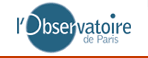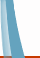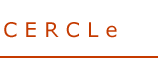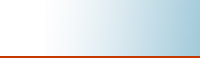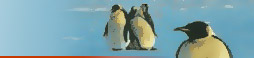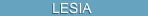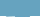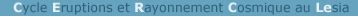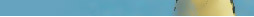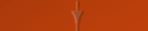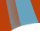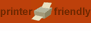[LAST VALUES]   [INTRO]   [COSMIC DATA REQUEST]   [GLE DATA REQUEST]   [RECENT POTENTIALS]

Recent values of the three principal modulation parameters

The three potentials considered here are:
• The heliocentric potential (O'Brien, 1976) which is used for example as an input for galactic cosmic ray dose calculation with the model CARI.
• The modulation potential (Nymmik et al., 1996). The energy spectrum of the different primary galactic particles and nuclei can be deduced from the modulation potential.
• The deceleration potential (Badhwar and O'Neill, 1996) which is for example an input of models such as EPCARD to calculate the radiation dose induced by galactic cosmic ray aboard aircrafts (see SIEVERT).
The following table gives the recent monthly modulation parameters : heliocentric and deceleration potentials are monthly average based on neutron monitor observations, and modulation potential, based on RI12 smoothed sunspot number, is averaged over 12 months and corresponds to a rigidity of 7 GV. Also given are the measured counts of the Kerguelen neutron monitor (used to calculate the heliocentric potential) and the estimated counts of the Climax monitor (used to calculate the deceleration parameter). This estimate is deduced with a linear regression method from Kerguelen observations (correlation coefficient = 0.987 and standard error of estimate = 43 for counts of the order of 4000).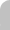Recent Potentials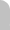month/year heliocentric* modulation* deceleration* measured** estimated*** potential potential potential Kerguelen counts Climax counts